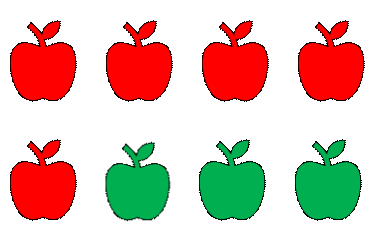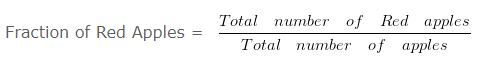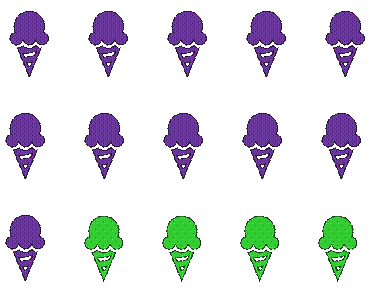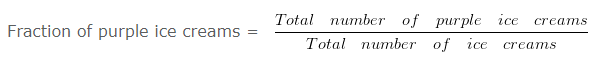# Fraction Representing the shaded Figure

Question 1

The Fraction that represents red colour apples, as a part of the total apples would be?Explanation

As we know that a Fraction represent a part of a whole.In the given figure,

Total number of Red apples = 5

Total number of Apples = 8

Therefore,

Fraction for the Red Apples = 5/8

Question 2

The Fraction that represents purple color ice creams, as a part of the total ice creams would be?Explanation

As we know that a Fraction represents a part of a whole.In the given figure,

Total number of purple ice creams = 11

Total number of ice creams = 15

Therefore,

Fraction for the purple ice creams = 11/15